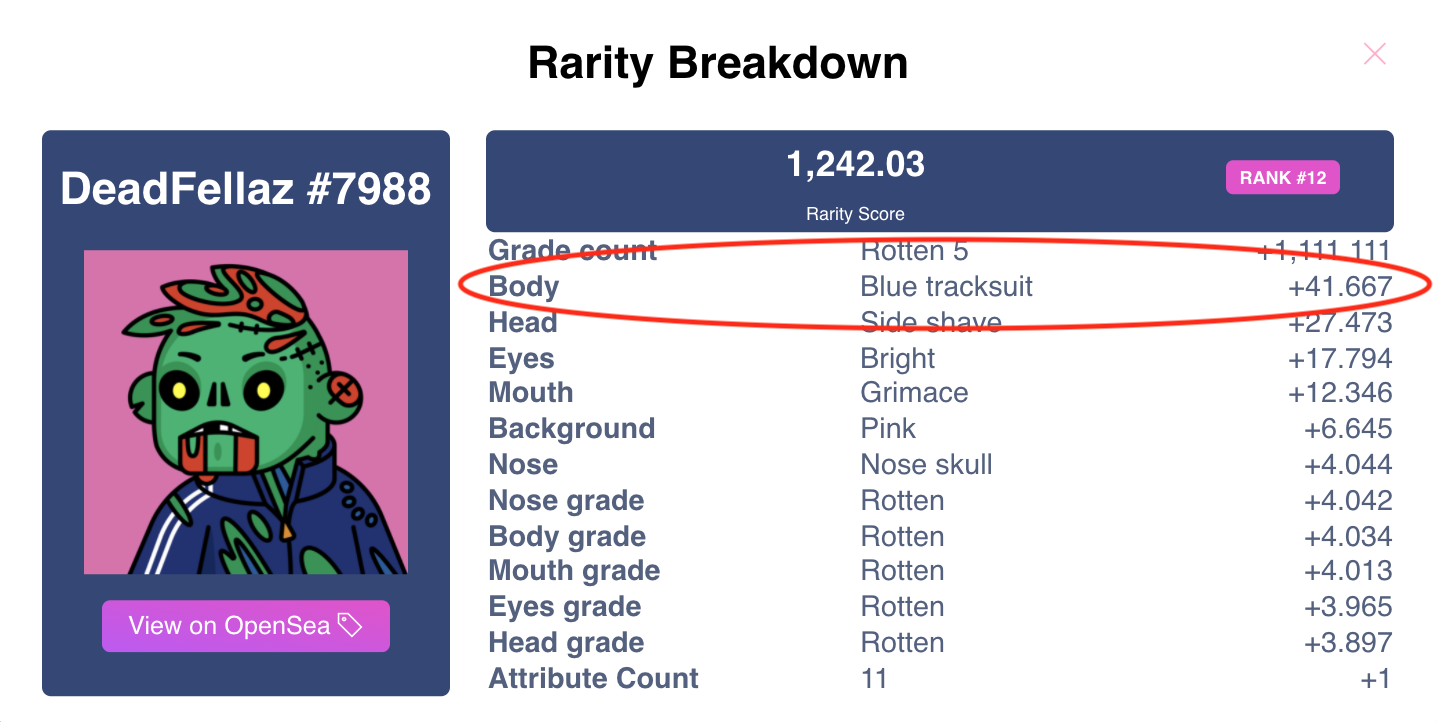# How do we calculate rarity?

Ranking rarity is more of an art than a science. Most rarity algorithms take into account how rare an individual trait is, which is good, but we thought that it was also important to include the rarity of a category in the score we calculate. Our rarity algorithm takes into account both how rare a trait is and how rare the category it's in is, as follows:

total = total number of NFTs in the collection

total_for_category = total number of NFTs in a particular category (ie. total number of Hats)

total_for_trait = total number of NFTs with a particular trait (ie. total number of Chefs Hats)

category_rarity = total / total_for_category

trait_rarity = total / total_for_trait

trait_rarity_score = sqrt(category_rarity) x trait_rarity

# ExampleLet's run through an example of calculating the rarity for a trait on this DeadFellaz. We will focus in on the Blue tracksuit attribute

First off, there are 10k total NFTs in the DeadFellaz collection, so total = 10000

There are also 10k total NFTs with a Body category, so total_for_category = 10000

There are 240 NFTs with a Blue tracksuit trait, so total_for_trait = 240

Now let's run the calculations

category_rarity = 10000 / 10000 = 1

trait_rarity = 10000 / 240 = 41.6667

trait_rarity_score = sqrt(1) * 41.6667 = 41.6667